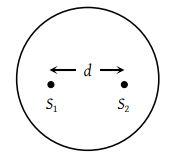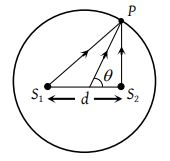# NEET Physics Wave Optics Questions Solved

Watch Physics > Wave Optics VideosTwo coherent sources separated by distance d are radiating in phase having wavelength λ. A detector moves in a big circle around the two sources in the plane of the two sources. The angular position of n = 4 interference maxima is given as(1) ${\mathrm{sin}}^{-1}\frac{n\lambda }{d}$

(2) ${\mathrm{cos}}^{-1}\frac{4\lambda }{d}$

(3) ${\mathrm{tan}}^{-1}\frac{d}{4\lambda }$

(4) ${\mathrm{cos}}^{-1}\frac{\lambda }{4d}$

(2) Here path difference at a point P on the circle is given by$\Delta x=d\mathrm{cos}\theta$….. (i)

For maxima at P

$\Delta x=n\lambda$….. (ii)

From equation (i) and (ii)

$n\lambda =d\mathrm{cos}\theta ⇒\theta {\mathrm{cos}}^{-1}\left(\frac{n\lambda }{d}\right)={\mathrm{cos}}^{-1}\left(\frac{4\lambda }{d}\right)$

Difficulty Level:

• 37%
• 36%
• 22%
• 7%
Crack NEET with Online Course - Free Trial (Offer Valid Till September 21, 2019)